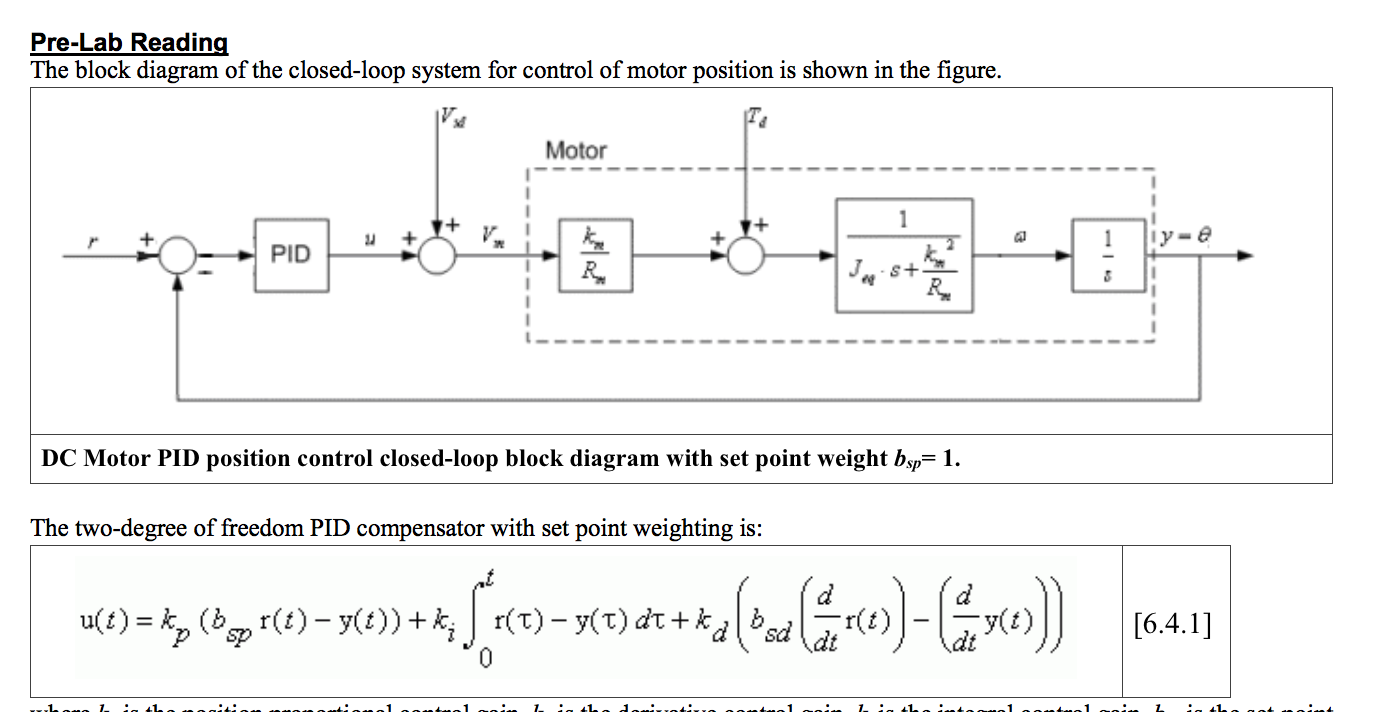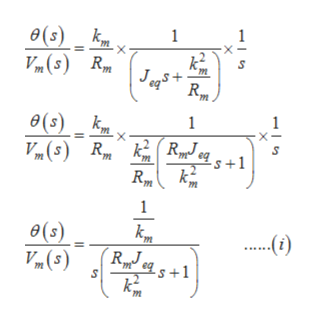# Pre-Lab ReadingThe block diagram of the closed-loop system for control of motor position is shown in the figure.Motor1Iy=e1PIDS+DC Motor PID position control closed-loop block diagram with set point weight bsp 1The two-degree of freedom PID compensator with set point weighting isu) ( () y(t)) +r(T)y(T) dtk(t)6.4.1]sd41-...

Question
109 views
1. Derive the plant transfer function, , for the DC motor position system in terms of the motor gain constant, K, and the time constant, τ.
2. Consider PD-Control with set-point weighting by setting ki = 0 in Equation 6.4.1.  Determine the closed-loop transfer function from the position reference, r, to the angular position output, θ in radians, i.e., Y(s)/R(s), in terms of K, τ, bsp, kp and kd.
3. Determine kp and kd in terms of the closed-loop natural frequency, ωn, damping ratio, ζ, motor time constant, τ, and motor gain constant, K.
4. Determine the steady-state error to a unit step input.  Given bsp = 1, determine the DC Gain of the closed-loop motor position system.help_outlineImage TranscriptionclosePre-Lab Reading The block diagram of the closed-loop system for control of motor position is shown in the figure. Motor 1 Iy=e 1 PID S+ DC Motor PID position control closed-loop block diagram with set point weight bsp 1 The two-degree of freedom PID compensator with set point weighting is u) ( () y(t)) + r(T)y(T) dtk (t) 6.4.1] sd 41-. .. fullscreen
check_circle

Step 1

Write the expression of the PID controller in Laplace transform:-

Step 2

The transfer function of the DC motor from the given block diagram can be expressed as:-help_outlineImage Transcriptionclose1 (s) mx Vm(s) R k Jegs m R 1 1 e(s) RyJ Rm eqs+1 ө(з) Vim(5) ....() k2 fullscreen
Step 3

The transfer function of the ...

### Want to see the full answer?

See Solution

#### Want to see this answer and more?

Solutions are written by subject experts who are available 24/7. Questions are typically answered within 1 hour.*

See Solution
*Response times may vary by subject and question.
Tagged in

### Electrical Engineering